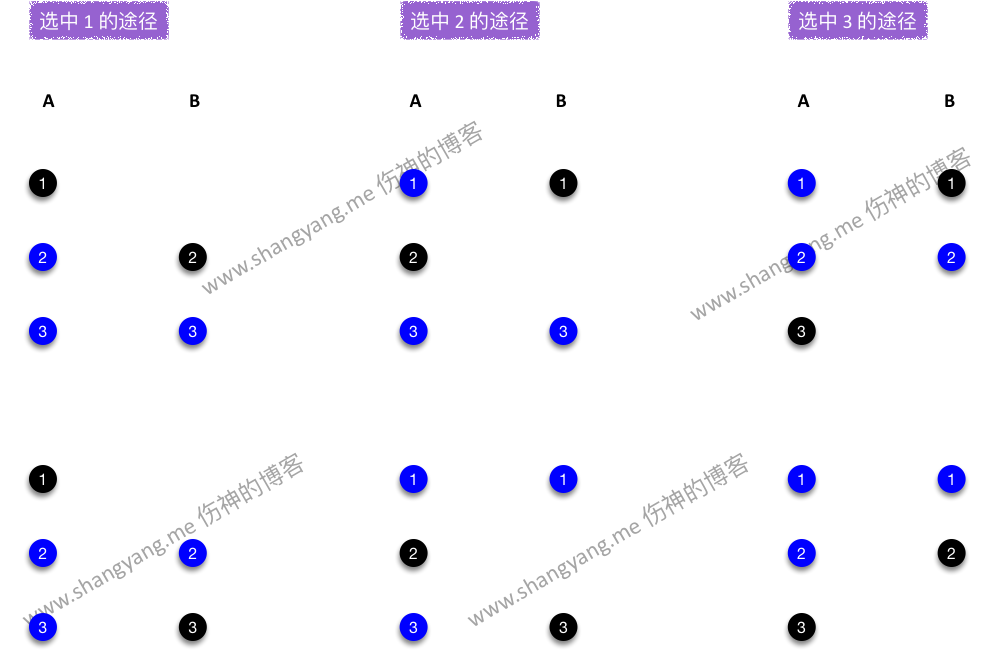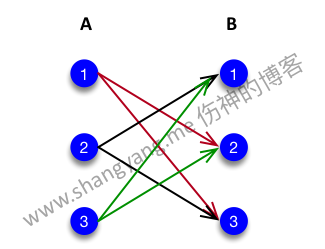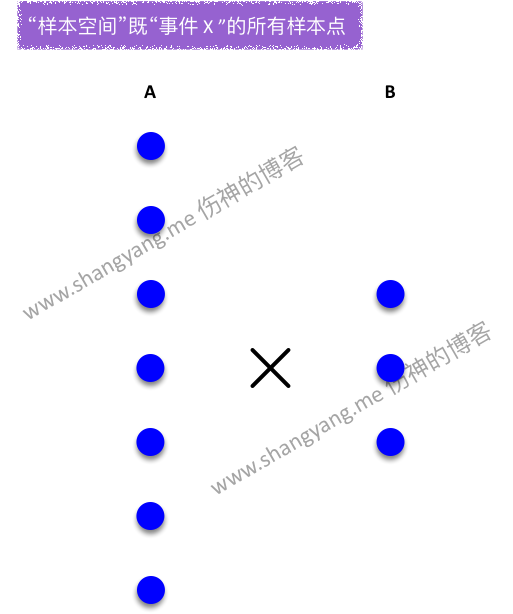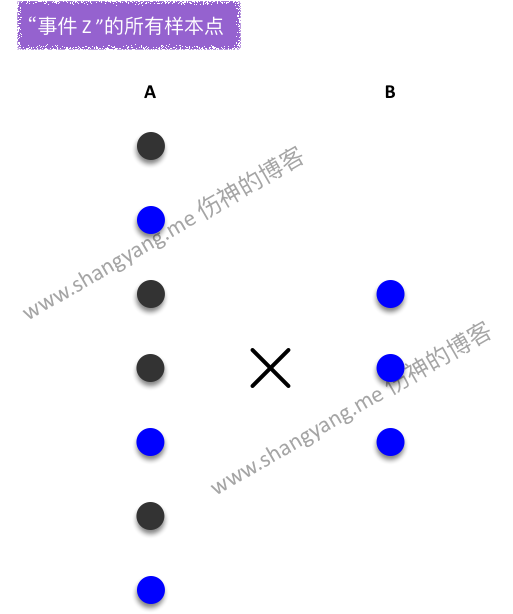# 加法原理和乘法原理

## 加法原理

1. 途径
指的是完成一件事情的某一条完整途径；比如从 A 点到 Z 点由多条途径（注意，加法原理需要保证途径是单向的），但是只要是有这么一条途径完成了 A -> Z，就称为一类途径；
2. $k$ 类不同路径
这里的类可以更为直观的理解成条，既是 $k$ 条路径；

## 乘法原理$$2 + 2 + 2$$

$$3 \times 2$$# 排列和组合

## 排列

$$P^r_n = n \times (n - 1) \times \ldots \times (n - r + 1) = \frac{n!}{(n-r)!}$$

## 组合

$$\binom{n}{r} = \frac{P^r_n}{r!} = \frac{n(n - 1) \dots (n - r + 1)}{r!} = \frac{n!}{r!\phantom{1}(n-r)!}$$

1. 如果取 2 次，假设，去重以后的样本点有 $m$ 个，其中任意一个样本点用 $E_x$ 表示，该样本点可表述为 { $E_{x1},\phantom{1}E_{x2}$ } 其中 $E_{x1},\phantom{1}E_{x2}$ 为该样本点所包含的元素，如果考虑样本点中元素先后取出的顺序，那么要计算出由该样本点所衍生出来的所有样本点（这里指的是交换元素的位置），根据乘法原理，就相当于对该样本点 { $E_{x1},\phantom{1}E_{x2}$ } 中的元素进行一次全排列既 $P_2$，所以我们得到，由样本点 { $E_{x1},\phantom{1}E_{x2}$ } 所衍生的所有样本点(包括自己) = $1 \times P_2$ = 2 个，由此我们推广至去重后的所有样本空间既 $m$ 个样本点，均满足该特性，又有，
➭ 首先，去重以后的样本空间既组合的样本空间 $= m = \binom{n}{r}$；
➭ 那么，根据上述的规律是否可以求得排列的样本空间呢？显然，排列的样本空间 $P^2_n = P_2 \times \binom{n}{2} = 2 \times \binom{n}{2}$；
➥ 由此，我们好像得到了一个非常重要的关系既排列的样本空间 = $P_r\phantom{1}\times$ 组合的样本空间( $r$ 表示取的次数 )；
➥ 但是，这个猜想是否适用于取任意 r 次呢？看下面的例证，

2. 如果取 r 次，同样假设，去重以后的样本点总共有 $m$ 个，其中任意一个样本点用 $E_x$ 表示，该样本点可表述为 {$E_{x1}, E_{x2}, \dots, E_{xr}$}，那么要求得该样本点所衍生的（通过修改元素先后取出的顺序）所有样本点就相当于对 {$E_{x1}, E_{x2}, \dots, E_{xr}$} 中的所有元素进行全排列既 $P^r_n$，同样，
➭ 首先，去重以后的样本空间既组合的样本空间 $= m = \binom{n}{r}$；
➭ 那么，显然，排列的样本空间 $P^r_n = P_r \times \binom{n}{r} = r! \times \binom{n}{r}$；
➥ 由此，该关系既排列的样本空间 = $P_r\phantom{1}\times$ 组合的样本空间( $r$ 表示取的次数 ) 成立；

$$P^r_n = r! \times \binom{n}{r}$$

# 实战

TODO，添加例子引用的出处；

## 抽样模型

1. 下图抽象表示事件 X 对应的所有样本点集合既样本空间的所有样本点，2. 下图抽象表示事件 Z 对应的所有样本点集合，灰色小球在事件 A 中本不应该出现，只是为了更为直观的与上图进行比对，笔者用灰色的小球表示哪些样本点减少了，可见事件 Z 在步骤 A 中可选的可能性减少了很多，因为只能从不合格品中去抽取，所以，事件 Z 的样本点相比于 $\Omega$ 而言减少了许多，也就是说其可能性减少了许多；

## 放回抽样

TODO…

1. 为什么概率的公式最后要乘以 $\binom{n}{m}$ ?
2. 为什么单单是对不合格品要进行一次组合？有太多的疑问需要解答，

1. 首先，解题之前，要知道这到底是一个排列还是一个组合的问题？从教材中的标准答案中，不难发现，将其视为了排列的问题，因为整体样本空间为 $N^n$；但是，笔者要说的是，上面的命题也完全可以被解读为组合问题，如果有个抄写员，将凡是含有相同合格品和不合格品的样本点均视为同一个样本点，那么就是一个重复组合的问题了，因为去重了既不考虑元素出现的先后次序了；所以，教材中的这个例子的描述含混不清，容易造成误解；既然该例子既可以被看做是重复排列又可以被看做是重复组合的问题，那么就顺着教材的观点分析吧，将其视为重复排列的问题；我猜想，教材中的出题人的出发点就是想探讨重复排列的问题；

2. 然后，首先还是来看“取出的 $n$ 个产品中恰好有 $1$ 个不合格品”的情况，将该事件命名为 $D_1$，取出 $n-1$ 个合格品重复排列的所有样本点 = $(N-M)^{n-1}$，取出 1 个不合格品的重复排列的所有样本点 = $M^1 = M$，那么现在的问题是，合格品的重复排列的样本空间和不合格品的重复排列的样本空间该如何合并在一起呢？首先，将抽取到 $n-1$ 个合格品的过程视为步骤 A，而将抽取到 1 不合格品的过程视为步骤 B，而根据前面的分析可知，步骤 A 的可能性为 $(N-M)^{n-1}$，而步骤 B 的可能性为 $M$，所以，根据乘法原理很容易想到，完成事件 $D_1$ 有 $(N-M)^{n-1} \times M$ 种可能；的确，根据乘法原理，这貌似就是最终结果了，但是别忘了，还有一种场景没考虑到，那就是步骤 A 的所有样本点与步骤 B 中的所有样本点之间是排列的关系，既是要考虑先后出现的次序，那么这层关系又该如何描述呢？在回答这个问题之前，笔者先通过下面这张概念图来模拟输出当前所得到的 $D_1’$ 的所有样本点，该集合是通过 $(N-M)^{n-1} \times M$ 计算得到的，如图，每一行表示 $D_1’$ 的一个样本点，图中模拟表示有 $(N-M)^{n-1} \times M$ 个样本点，一个样本点中的元素包含两个部分，$n-1$ 个合格品和和 $1$ 个不合格品；通过这张图，一下子就可以发现问题的症结所在了，针对每一个样本点而言，红色的小点不应该只出现在右边，我们要考虑次序，也就是说红色的小球可以出现在一个样本点中的任意的一个位置，所以，我们漏掉了这种可能性，那么这种可能性又该如何计算呢？最直观的解决方案是，针对上图中的任意一个样本点，将其红色小球插入任意一个黑色小球的左右两边之后所能够得到的所有可能性(补充，为什么这里解决问题的思路是插入而不是将它们进行一次全排列呢？因为 $D_1’$ 中的每一个样本点都是由排列过后的合格品和非合格品的样本点所组成的，它们的顺序早已排列过了，因此 $D_1’$ 中任意一个样本点中黑色小球的顺序和红色小球的顺序是固定的，不可变的)，很显然，红色小球能够插入的位置有 $n$ 个，因此有 $\binom{n}{1} = n$ 中可能性；又因为该可能性适合于 $D_1’$ 中所有的样本点，所以得到最终事件的样本点集合 $D_1 = (N-M)^{n-1} \times M^1 \times \binom{n}{1}$ ；Ok，这里便回答了我的第一个问题；但是第二个问题呢？为什么解决问题的思路必须从不合格品入手呢？实际上，同样可以从合格品上入手思考解决思路，也可以将 $n-1$ 个合格品插入$1$ 个不合格品的两边，这种情况可以理解为，总共有 n 个位置，但是只随机拿出 $n-1$ 个位置给你安放合格品，另外的 $1$ 个随机的位置安放不合格品，因此这种插入的方式有 $\binom{n}{n-1}$ 种可能，所以从这种角度思考计算所得到事件 $D_1$ 的样本点集合 $D_1=(N-M)^{n-1} \times M^1 \times \binom{n}{n-1}$ ；又因为 $\binom{n}{n-1} = \frac{n!}{(n-1)!} = n = \binom{n}{1}$，因此实际上 $(N-M)^{n-1} \times M^1 \times \binom{n}{n-1} = (N-M)^{n-1} \times M^1 \times \binom{n}{1}$，所以实际上两种不同的插入方式所得到结果是相同的，至此，第二个问题得到了完美解答。这下心里感觉好多了 :) 棒棒哒！！！

3. 最后，来看“取出的 $n$ 个产品中恰好有 $m$ 个不合格品”的情况，将该事件命名为 $D_m$，同理，通过将抽取不合格品 A 和抽取合格品 B，我们可以得到 $D_m’ = M^m \times (N-M)^{n-m}$，同样，笔者通过下面这张概念图来描述当前样本点集合的情况，如图，每一行表示一个样本点，红色小球表示 $m$ 个不合格品，黑色小球表示 $n-m$ 个合格品，剩下的工作同样是要对每一个样本点中的合格品和不合格品进行插入操作才能得到最终的样本点集合 $D_m$，插入动作有两种方式，可以是将红色小球既不合格品插入黑色小球既合格品中，也可以是将黑色小球插入红色小球中；两种方式的解决思路其实都可以归纳为，有 $n$ 个空位，先拿一部分的空位安放第一类元素，剩下的空位安放第二类元素，并且不考虑元素安放的先后顺序，那么只要计算出第一类元素可被安放的所有可能，既是这两类元素同时被安放的所有可能；

先安放不合格品

$n$ 个空位，随机安放 $m$ 个不合格品，不考虑 $m$ 个合格品的顺序，同时也不考虑 $n-m$ 个合格品安放的顺序，那么安放 $m$ 个不合格品的可能性是一个组合问题，该可能性为 $\binom{n}{m}$，而该可能性适合于 $D_m$ 中的每一个样本点，所以，最终的事件 $D_m$ 的样本点集合为 $$D_m = \binom{n}{m} \times M^m \times (N-M)^{n-m}$$

先安放合格品

同理，如果从先安放合格品的角度出发，可以得到可安放的可能性为 $\binom{n}{n-m}$，而很容易证明 $\binom{n}{n-m} = \binom{n}{m}$，所以实际上无论从先安放合格品还是先安放不合格品出发，得到的可能性都是一样的，因此最终得到事件 $D_m$ 的样本点集合也都是一样的。

# 技巧

## 某个步骤的可能性及其概率

1. 当某个步骤明确只取一个样本点的时候，这个时候该步骤的可能性(既可取的样本点)为 1，
比如掷一次骰子，那么该步骤的可能性为 1，假设骰子的点数总共有 6 种可能，因此事件的样本空间总共含有 6 个样本点；因此概率为 ${1\over 6}$。

2. 当某个步骤的表述方式为任取一个某种类型的样本点的时候，假设这种类型的样本点为 n 个，那么该步骤的可能性(既可取的样本点)为 n；
假设该步骤的其它所有类型的样本点为 m，因此样本空间的所有样本点为 $m+n$，因此概率为 ${n\over m+n}$；

## 特殊事件的样本点集合或样本空间

### 一对多模型

《概率论与数理统计教程》 第二版，茆诗松版